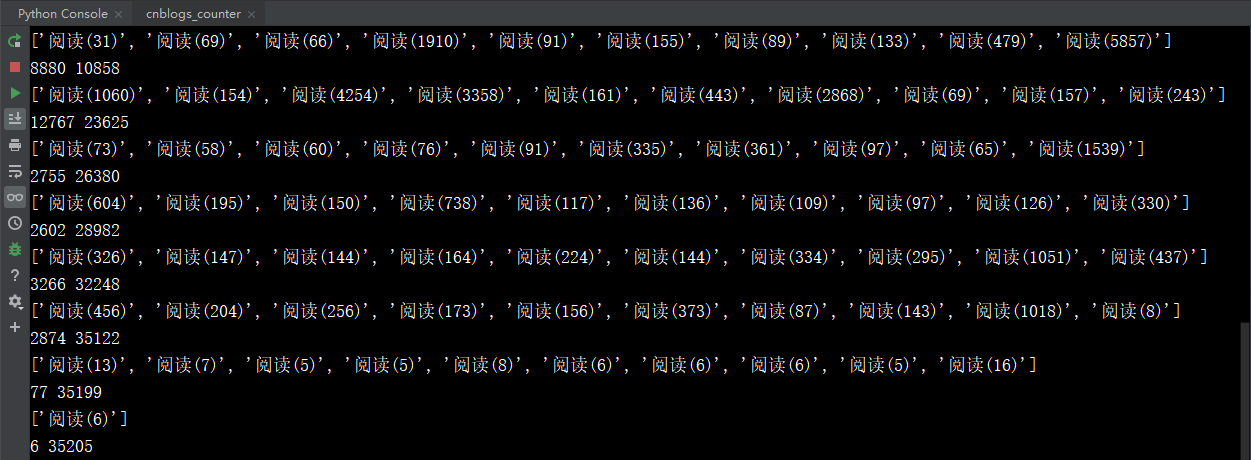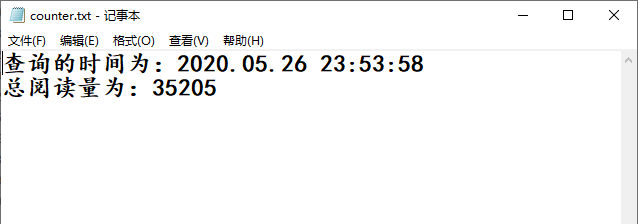﻿ Python实现统计文章阅读量的方法详解_python_脚本之家
python# Python实现统计文章阅读量的方法详解

## 实现代码

```#导入需要的模块
import time
import requests
from lxml import etree

#进行UA伪装
"User-agent":"Mozilla/5.0 (Windows NT 10.0; Win64; x64) AppleWebKit/537.36 (KHTML, like Gecko) Chrome/79.0.3945.130 Safari/537.36"
}
#创建文件，存储爬到的数据，做持久化,以追加的方式打开，可读可写
fp=open('./counter.txt','a+',encoding = 'utf-8')
#记录当前查询时间
select_time=time.strftime('%Y.%m.%d %H:%M:%S ',time.localtime(time.time()))
fp.write('查询的时间为：'+select_time+'\n')
def spider():
#总阅读量
counts=0
#分页爬取数据加和
for page_num in range(1,20):
#设置url
url="https://www.cnblogs.com/andrew3/default.html?page=%d"
new_url=format(url%page_num)
#获取页面数据
tree=etree.HTML(page_text)
#解析数据，得到每页每篇博文的阅读量
count_list=tree.xpath('//div[@class="forFlow"]/div/div[@class="postDesc"]/span/text()')
print(count_list)
#每一页的阅读量
sum=0
#计算每页的阅读量
for i in range(len(count_list)):
sum+=int(count_list[i][3:len(count_list[i])-1])
#计算总阅读量
counts+=sum
#打印每页每篇博文的阅读量
print(sum,counts)
#总阅读量持久化
fp.write("总阅读量为：%s" % counts + '\n')
#关闭文件，释放资源
def close_file():
fp.close()
def main():
spider()
close_file()
if __name__ == '__main__':
main()```

## 效果图